Courses

NCERT Solutions - Chapter 14: Statistics, Class 10, Maths(Part 3) Notes - Class 10

Class 10: NCERT Solutions - Chapter 14: Statistics, Class 10, Maths(Part 3) Notes - Class 10

The document NCERT Solutions - Chapter 14: Statistics, Class 10, Maths(Part 3) Notes - Class 10 is a part of Class 10 category.
All you need of Class 10 at this link: Class 10

Exercise 14.3

Q1 :
The following frequency distribution gives the monthly consumption of electricity of 68 consumers of a locality. Find the median, mean and mode of the data and compare them.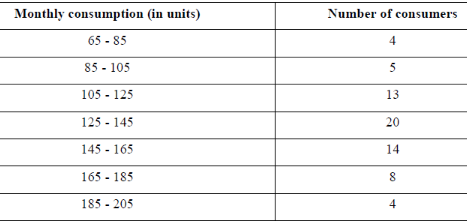To find the class marks, the following relation is used.

Class Mark= (upper class limit + lower class limit)/2

Taking 135 as assumed mean (a), di, ui,fiuare calculated according to step deviation method as follows.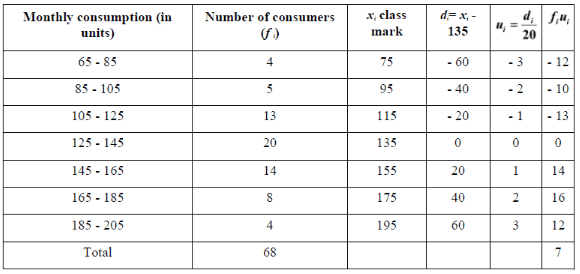From the table, we obtain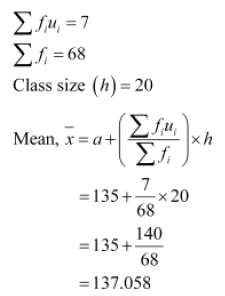From the table, it can be observed that the maximum class frequency is 20, belonging to class interval 125 - 145.
Modal class = 125 - 145
Lower limit (l) of modal class = 125
Class size (h) = 20
Frequency (f1) of modal class = 20
Frequency (f0) of class preceding modal class = 13
Frequency (f2) of class succeeding the modal cla

Q2 :
If the median of the distribution is given below is 28.5, find the values of x and y.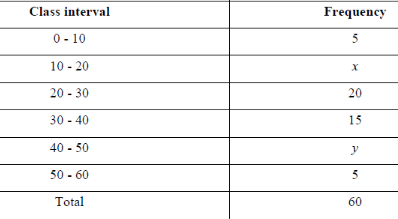The cumulative frequency for the given data is calculated as follows.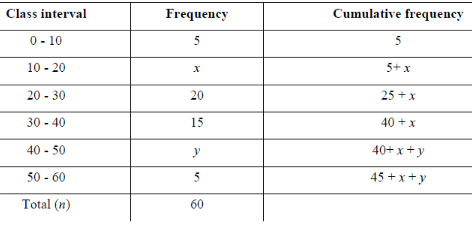From the table, it can be observed that n = 60
45 + x + y = 60
x + y = 15 (1)
Median of the data is given as 28.5 which lies in interval 20 - 30.
Therefore, median class = 20 - 30
Lower limit (l) of median class = 20
Cumulative frequency (cf) of class preceding the median class = 5 + x

Frequency (f) of median class = 20
Class size (h) = 10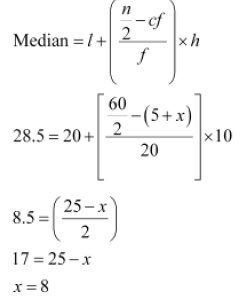From equation (1),
8 + y = 15
y = 7
Hence, the values of x and y are 8 and 7 respectively.

Q3 :
A life insurance agent found the following data for distribution of ages of 100 policy holders. Calculate the median age, if policies are given only to persons having age 18 years onwards but less than 60 year.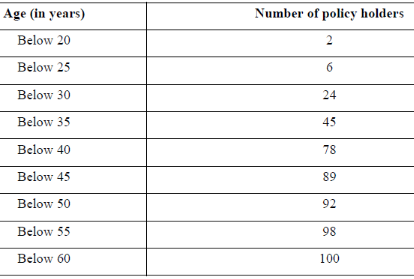Here, class width is not the same. There is no requirement of adjusting the frequencies according to class intervals. The given frequency table is of less than type represented with upper class limits. The policies were given only to persons with age 18 years onwards but less than 60 years. Therefore, class intervals with their respective cumulative frequency can be defined as below.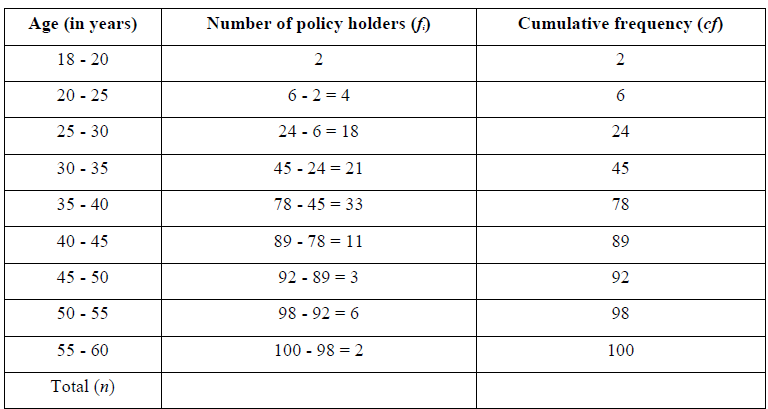From the table, it can be observed that n = 100.
Cumulative frequency (cf) just greater than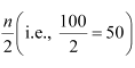is 78, belonging to interval 35 - 40.
Therefore, median class = 35 - 40
Lower limit (l) of median class = 35
Class size (h) = 5
Frequency (f) of median class = 33
Cumulative frequency (cf) of class preceding median class = 45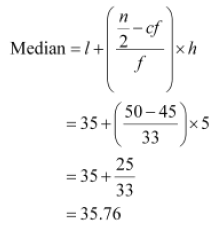Therefore, median age is 35.76 years.

Q4 :
The lengths of 40 leaves of a plant are measured correct to the nearest millimeter, and the data obtained is represented in the following table
: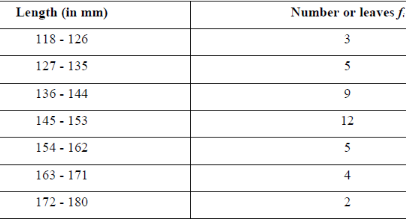Find the median length of the leaves.
(Hint: The data needs to be converted to continuous classes for finding the median, since the formula
assumes continuous classes. The classes then change to 117.5 - 126.5, 126.5 - 135.5… 171.5 - 180.5)
The given data does not have continuous class intervals. It can be observed that the difference between two class
intervals is 1. Therefore, 1/2 = 0.5  has to be added and subtracted to upper class limits and lower class limits
respectively.
Continuous class intervals with respective cumulative frequencies can be represented as follows.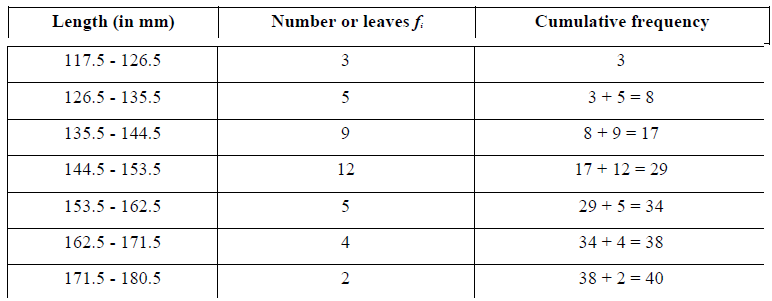From the table, it can be observed that the cumulative frequency just greater than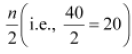is 29,
belonging to class interval 144.5 - 153.5.
Median class = 144.5 - 153.5
Lower limit (l) of median class = 144.5
Class size (h) = 9
Frequency (f) of median class = 12
Cumulative frequency (cf) of class preceding median class = 17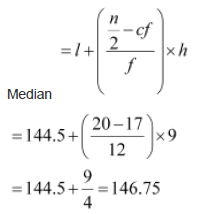Therefore, median length of leaves is 146.75 mm.

Q5 :
Find the following table gives the distribution of the life time of 400 neon lamps: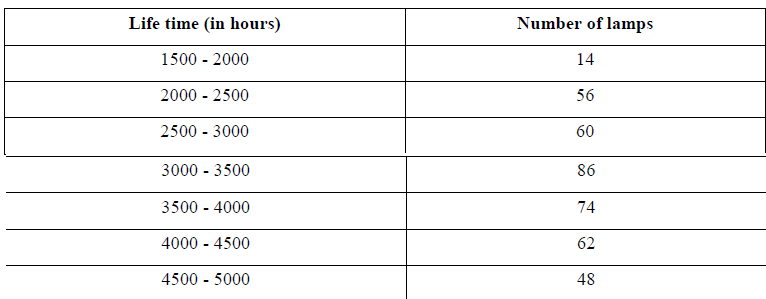Find the median life time of a lamp.

Thecumulative frequencies with their respective class intervals are as follows.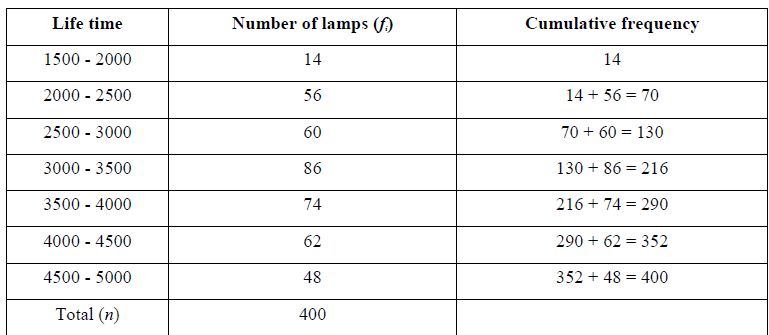It can be observed that the cumulative frequency just greater than is 216, belonging to class
interval 3000 - 3500.
Median class = 3000 - 3500
Lower limit (l) of median class = 3000
Frequency (f) of median class = 86
Cumulative frequency (cf) of class preceding median class = 130
Class size (h) = 500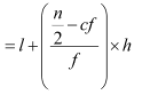Median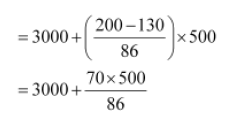= 3406.976
Therefore, median life time of lamps is 3406.98 hours.

Q6 :
100 surnames were randomly picked up from a local telephone directory and the frequency distribution of the number of letters in the English alphabets in the surnames was obtained as follows: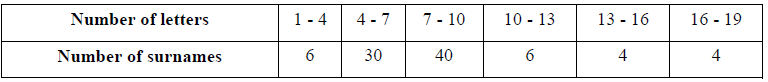Determine the median number of letters in the surnames. Find the mean number of letters in the surnames?
Also, find the modal size of the surnames.

The cumulative frequencies with their respective class intervals are as follows.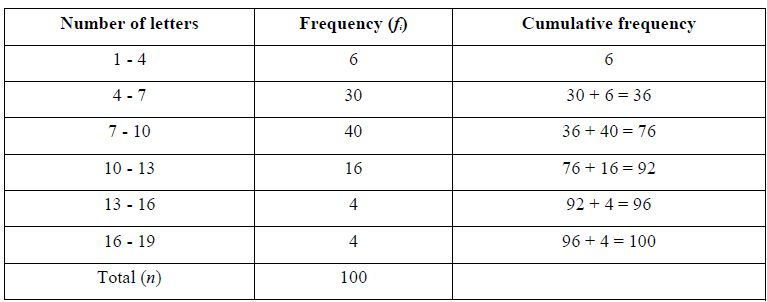It can be observed that the cumulative frequency just greater than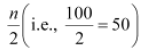is 76, belonging to class
interval 7 - 10.
Median class = 7 - 10
Lower limit (l) of median class = 7
Cumulative frequency (cf) of class preceding median class = 36
Frequency (f) of median class = 40

Class size (h) = 3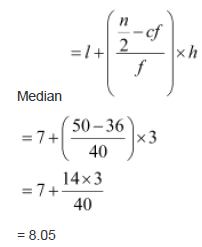To find the class marks of the given class intervals, the following relation is used.

Class Mark= (upper class limit + lower class limit)/2

Taking 11.5 as assumed mean (a), di, ui,and fiui are calculated according to step deviation method as follows.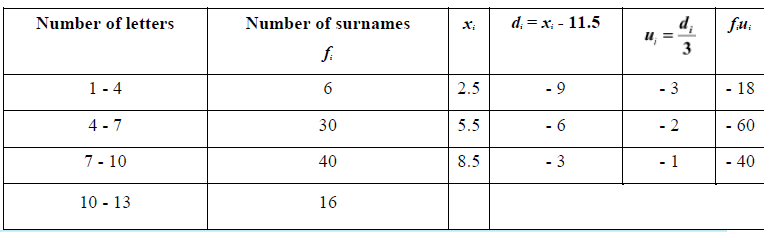Q7 :
The distribution below gives the weights of 30 students of a class. Find the median weight of the students.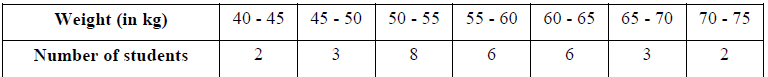The cumulative frequencies with their respective class intervals are as follows.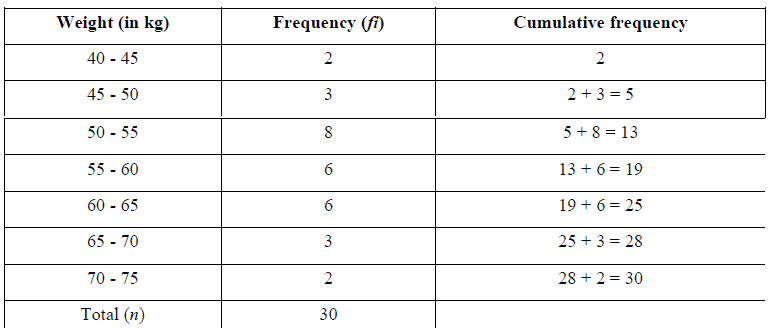Cumulative frequency just greater than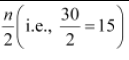is 19, belonging to class interval 55 - 60.
Median class = 55 - 60
Lower limit (l) of median class = 55
Frequency (f) of median class = 6
Cumulative frequency (cf) of median class = 13
Class size (h) = 5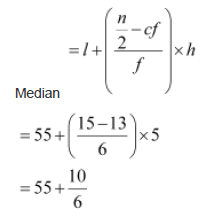= 56.67
Therefore, median weight is 56.67 kg.

Exercise 14.4 :

Q1 :
The following distribution gives the daily income of 50 workers of a factory.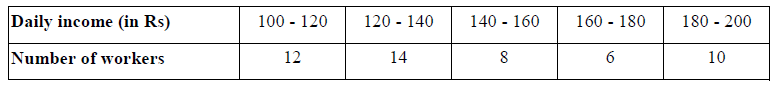Convert the distribution above to a less than type cumulative frequency distribution, and draw its ogive.

The frequency distribution table of less than type is as follows.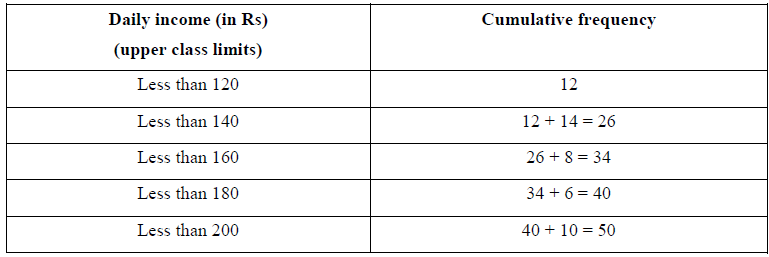Taking upper class limits of class intervals on x-axis and their respective frequencies on y-axis, its ogive can be drawn as follows.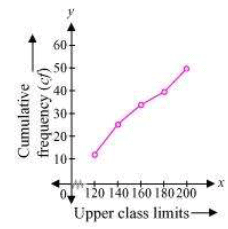Q2 :
During the medical check-up of 35 students of a class, their weights were recorded as follows: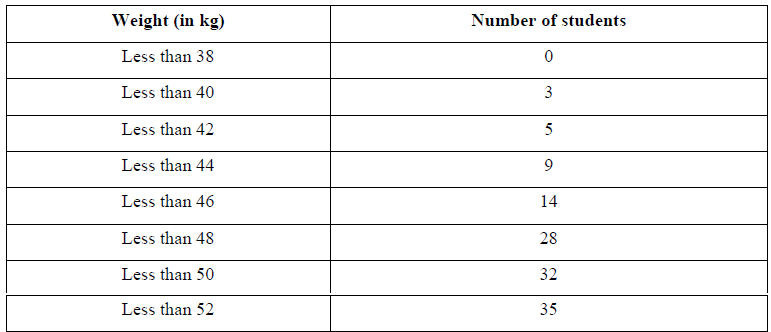Draw a less than type ogive for the given data. Hence obtain the median weight from the graph verify the result by using the formula.

The given cumulative frequency distributions of less than type are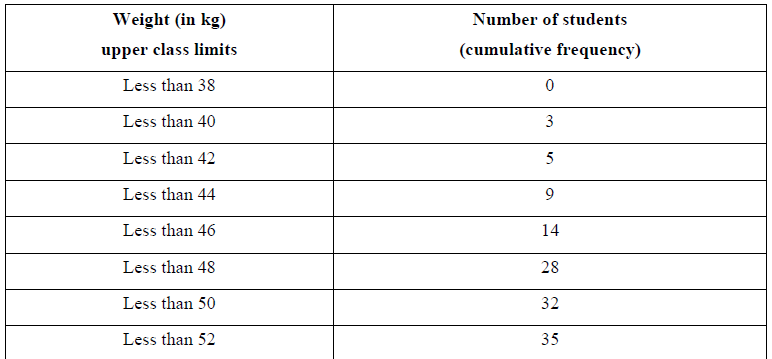Taking upper class limits on x-axis and their respective cumulative frequencies on y-axis, its ogive can be drawn as follows.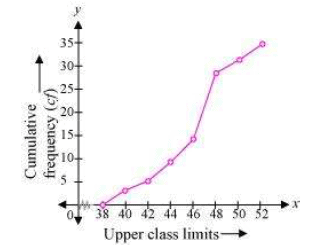Here, n = 35
So,n/2 = 17.5
Mark the point A whose ordinate is 17.5 and its x-coordinate is 46.5. Therefore, median of this data is 46.5.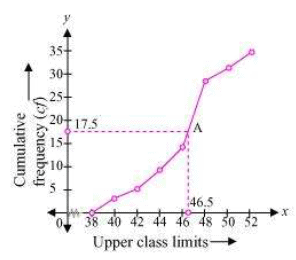It can be observed that the difference between two consecutive upper class limits is 2. The class marks with their respective frequencies are obtained as below.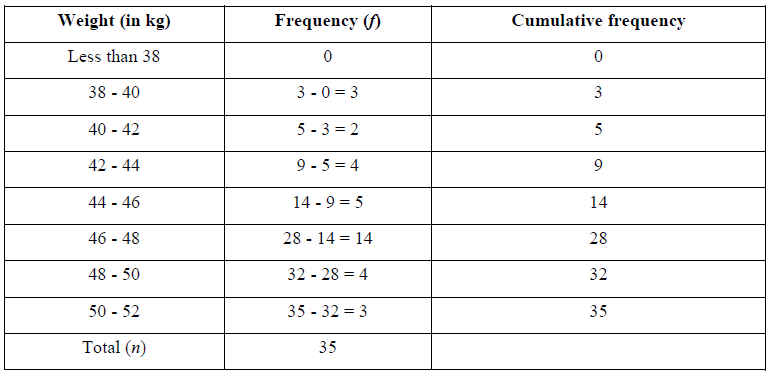The cumulative frequency just greater than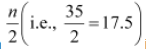is 28, belonging to class interval 46 - 48.
Median class = 46 - 48
Lower class limit (l) of median class = 46
Frequency (f) of median class = 14
Cumulative frequency (cf) of class preceding median class = 14
Class size (h) = 2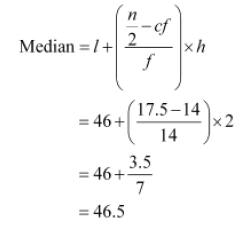Q3 :
The following table gives production yield per hectare of wheat of 100 farms of a village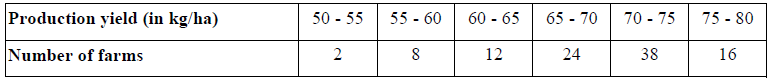Change the distribution to a more than type distribution and draw ogive.

The cumulative frequency distribution of more than type can be obtained as follows.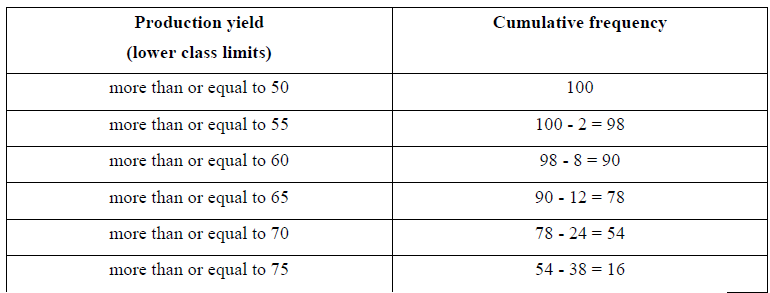Taking the lower class limits on x-axis and their respective cumulative frequencies on y-axis, its ogive can be
obtained as follows.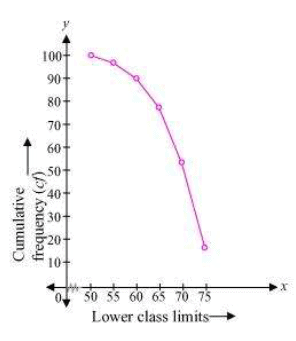The document NCERT Solutions - Chapter 14: Statistics, Class 10, Maths(Part 3) Notes - Class 10 is a part of Class 10 category.
All you need of Class 10 at this link: Class 10Use Code STAYHOME200 and get INR 200 additional OFF Use Coupon Code

Top Courses for Class 10Top Courses for Class 10Track your progress, build streaks, highlight & save important lessons and more!

,

,

,

,

,

,

,

,

,

,

,

,

,

,

,

,

,

,

,

,

,

,

,

,

,

,

,

;